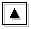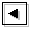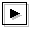## 1. Introduction### 1.2 Aims of this study

Although the physical interpretations for the most unstable modes were given by TI2006, other unstable modes, neutral modes, and continuos modes are not well examined. The following problems are left unexamined:

• Equatorial Rossby modes (Matsuno, 1966) are not observed in the results of TI2006, although equatorial Kelvin modes, inertial gravity modes, and mixed Rossby-gravity modes were identified. TI2006 speculated that all equatorial Rossby modes assimilate into continuous modes. However, the structural change of continuous modes with such assimilation are not shown.
• Among symmetric unstable modes, TI2006 considered only the lowest order modes which correspond to so-called inertially unstable mode. The resonating neutral modes causing high-order symmetric unstable modes were not clarified.
• In some dispersion curves obtained by TI2006, nearby dispersion curves of neutral modes intersect (an example is shown in figure (c) in table 1). TI2006 does not discuss the nature of such neutral modes.
• The dispersion curves of equatorial Kelvin modes and westward mixed Rossby-gravity modes are obscure when the two modes resonate. Their dispersion curves are buried among dispersion curves of continuous modes in the figures of TI2006.

In this paper, the above mentioned problems are examined in order to give interpretations for all modes obtained by TI2006. Each problem is examined in the following each section. In section 2, governing equations are described. In sections 3 to 6, more detailed description for each problem and results are given. Section 7 is a summary.